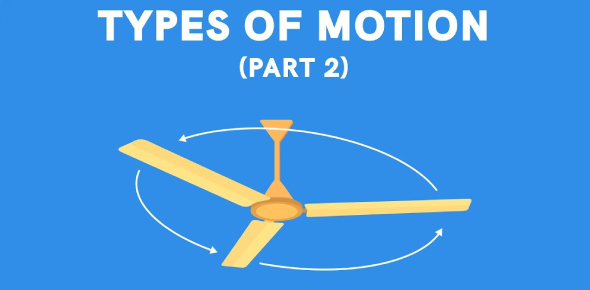# Physics Quiz: Introduction To Motion! Trivia Questions

15 Questions | Total Attempts: 159Settings.

• 1.
Motion
• A.

Path along which an object moves; straight or curve

• B.

Motion of an object by displaying its location at various equally spaced times on the same diagram

• C.

Change of an objects position with time

• D.

Motion of an object along a trajectory

• 2.
Trajectory
• A.

Path along which an object moves; straight or curve

• B.

An arrow drawn from the origin to an objects position

• C.

Motion of an object along a trajectory

• D.

Motion of an object by displaying its location at various equally spaced times on the same diagram

• E.

Change of an objects position with time

• 3.
Motion diagram
• A.

Motion of an object along a trajectory

• B.

An arrow drawn from the origin to an objects position

• C.

Change of position

• D.

An object represented as a mass at a single point

• E.

Motion of an object by displaying its location at various equally spaced times on the same diagram

• 4.
Translational motion
• A.

Motion of an object by displaying its location at various equally spaced times on the same diagram

• B.

Motion of an object along a trajectory

• C.

Motion of an object represented as a mass concentrated at a single point

• D.

Path along which an object moves; straight or curve

• E.

An arrow drawn from the origin to an objects position.

• 5.
Particle
• A.

An object represented as a mass at a single point

• B.

Physical quantity described by a single number (with unit).

• C.

Motion of an object along a trajectory

• D.

Motion of an object by displaying its location at various equally spaced times on the same diagram

• E.

An arrow drawn from the origin to an objects position

• 6.
Particle model
• A.

Motion of an object along a trajectory

• B.

An object represented as a mass at a single point

• C.

Change of position

• D.

Motion of an object represented as a mass concentrated at a single point

• E.

An arrow drawn from the origin to an objects position

• 7.
Position Vector
• A.

Change of an objects position with time

• B.

Change of position

• C.

An arrow drawn from the origin to an objects position.

• D.

An object represented as a mass at a single point

• E.

Motion of an object along a trajectory

• 8.
Scalar Quantity
• A.

Motion of an object along a trajectory

• B.

Physical quantity described by a single number (with unit).

• C.

Quantity having both a size (far, fast) and a direction (left or right, north or south ext).

• D.

An arrow drawn from the origin to an objects position

• E.

A continuous line or curve showing an objects position as a function of time

• 9.
Vector Quantity
• A.

Quantity having both a size (far, fast) and a direction (left or right, north or south ext).

• B.

Motion of an object along a trajectory

• C.

Physical quantity described by a single number (with unit).

• D.

An arrow drawn from the origin to an objects position

• E.

A continuous line or curve showing an objects position as a function of time

• 10.
Displacement
• A.

A continuous line or curve showing an objects position as a function of time

• B.

An arrow drawn from the origin to an objects position

• C.

Change of position

• D.

Motion of an object along a trajectory

• E.

Motion of an object by displaying its location at various equally spaced times on the same diagram

• 11.
Position vs Time graph
• A.

Has a continuous line or curve showing an objects position as a function of time

• B.

Has a continuous line or curve showing the objects time as a function of position

• 12.
Average Acceleration can be calculated as...
• A.

(distance)/ (time interval)

• B.

(change of velocity)/ (time interval)

• C.

(time interval)/ ( change of velocity)

• D.

(time interval)/ (distance)

• 13.
Average Velocity can be calculated as ...
• A.

(change of velocity)/ (time interval)

• B.

(time interval)/ ( change of velocity)

• C.

(time interval)/ (distance)

• D.

(distance)/ (time interval)

• 14.
How to do a Pictorial representation. choose all that apply
• A.

Draw Motion Diagram

• B.

Establish coordinate system

• C.

Sketch the situation

• D.

Define Symbols

• E.

List known information and Identify the desired Unknowns

• 15.
Significant figures. choose the correct answers
• A.

.0020 has two significant figures

• B.

6.20 has three significant figures

• C.

10.020 has four significant figures

• D.

0.0 has two significant figures

• E.

.00001 has 5 significant figures

Related TopicsBack to top